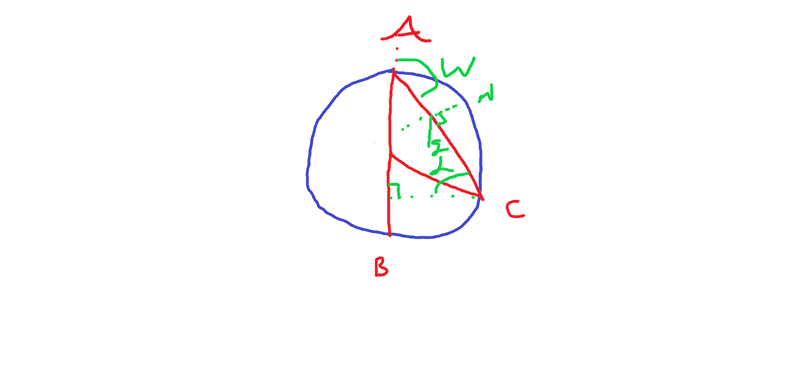# Bead sliding on a Vertical Circular Loop versus in Free Fall

Rubberduck2005
Homework Statement:
A circle with a radius R lies in the vertical plane. A bead falling under gravity moves from A( the top point) to B ( an antipodal point to A), Moving a long the diameter. Now consider a separate bead, such a bead leaves A at an angle W from the vertical and moves a long a cord to a point C. Prove that both beads take the same time to complete their respective journeys.
Relevant Equations:
g=9.81ms^-2 j is the y unit vector , i the x unit vector
I can evaluate the first beads motion easily A to B is -2Rj considering the point B as y=0 the motion of the bead will be -gt^2/2+2R=0 which implies t=2√(R/G) , this is ok but what I am struggling with is A to C I can see that the angle between the beads weight and it's negative normal force is W-90 as (180=180-W + 90 + (the new angle=L), given this I can derivate that the acceleration moving down the plane is gsin(L)=gsin(W-90)=-gcos(W) now the motion of this bead need be -1/2(gcos(w))t^2+2R=C(J) I can also see that A to C= A to O + O to C= -R + O to C, I can't find the Y coordinated of C. If you see a flaw in my reason please point it out.

### ​

Rubberduck2005Homework Helper
Gold Member
2022 Award
the angle between the beads weight and it's negative normal force is W-90
I get 270-W.
can't find the Y coordinated of C
What is angle BOC in terms of BAC? (Well known theorem.)

Rubberduck2005
haruspex With regards to your first comment I create a triangle using (180 -W) as my top angle and the bottom left being 90 from this I deduced that the angle in the bottom left need be 90-W thus the angle between the weight and the negative normal be that angle?

Rubberduck2005
@haruspex I am also not sure what theorem you are talking about

Homework Helper
Gold Member
2022 Award
the angle in the bottom left
I assume you mean bottom right, and specifically the angle that AC makes to the horizontal. That is not what I thought you meant by the angle between the beads weight and it's negative normal force. I took that to mean the angle between a vertical line down from the particle and the upward normal to AC from the particle.
@haruspex I am also not sure what theorem you are talking about
Angles subtended by a chord at centre and circumference.
Google that if you still don't know.

Homework Helper
Gold Member
angle BOC = 2BAC-180?@haruspex
Let's see if that works when OC is horizontal, in which case BOC = 90o and BAC = 45o. If your formula is correct, it should be true that 90o = 2×45o - 180o. Is it true? If not, what does this simple test suggest about the correct formula?

Rubberduck2005
kuruman hi thank you for dismissing my claim can you link me to a video on this theorem or simply hint at how to achieve insight into what the idea could be

Rubberduck2005
angles of the same base cord are equal? but it doesn't connect with the circumforance

Homework Helper
Gold Member
2022 Award
angles of the same base cord are equal? but it doesn't connect with the circumforance

The first that came up when I googled was https://www.cuemath.com/geometry/arcs-and-subtended-angles, though it refers to angles subtended by arcs rather than chords. Same deal.
A little further on I hit https://amsi.org.au/teacher_modules/Circle_Geometry.html#Angles_at_the_centre_and_circumference.
Immediately following, https://www.scienceandmathsrevision.co.uk/topic/circle-theorems/.
How did you not find a relevant one?

Homework Helper
Gold Member
Your schematic is a bit off regarding how W angle is defined: The problem statement clearly says that W is the angle from the vertical while in your schematic you show it to be the angle from the horizontal.

If we define W as above then the component of acceleration parallel to AC is simply ##g\cos W##.
Then I used cosine law to find AC as a function of the radius R and the angle AOC. Then used a bit of trigonometry and geometry (namely that the angles OAC(=W by definition) and OCA are equal (why?), hence AOC=180-2W) to transform the expression for AC. Then simply used $$\frac{1}{2}g\cos W t^2=AC$$ and I got the desired result.

Homework Helper
Gold Member
2022 Award
while in your schematic you show it to be the angle from the horizontal.
As I read the drawing, it is the angle to the upward vertical, so obtuse.

•Rubberduck2005
Homework Helper
Gold Member
As I read the drawing, it is the angle to the upward vertical, so obtuse.
Ah yes, I see that now. Well , anyway, in my opinion it works better if we define W=OAC.

Homework Helper
Gold Member
I think this problem is more of a problem in trigonometry and geometry, from physics the only equation we use is the equation of uniform accelerating motion in a line, that is##s=\frac{1}{2}at^2##.

•kuruman
•